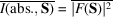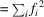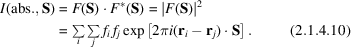International
Tables for
Crystallography
Volume F
Crystallography of biological macromolecules
Edited by E. Arnold, D. M. Himmel and M. G. Rossmann

International Tables for Crystallography (2012). Vol. F, ch. 2.1, pp. 56-57   | 1 | 2 |

## Section 2.1.4.6. The structure factor

J. Drentha*

aLaboratory of Biophysical Chemistry, University of Groningen, Nijenborgh 4, 9747 AG Groningen, The Netherlands
Correspondence e-mail: j.drenth@chem.rug.nl

#### 2.1.4.6. The structure factor

| top | pdf |

For noncentrosymmetric structures, the structure factor,is an imaginary quantity and can also be written as2It is sometimes convenient to split the structure factor into its real part, A(S), and its imaginary part, B(S). For centrosymmetric structures,if the origin of the structure is chosen at the centre of symmetry.

The average value of the structure-factor amplitudedecreases with increasingor, because, with increasing reflecting angle θ.

This is caused by two factors:

 (1) A stronger negative interference between the electrons in the atoms at a larger scattering angle; this is expressed in the decrease of the atomic scattering factor as a function of S. (2) The temperature-dependent vibrations of the atoms. Because of these vibrations, the apparent size of an atom is larger during an X-ray exposure, and the decrease in its scattering as a function of S is stronger. If the vibration is equally strong in all directions, it is called isotropic, and the atomic scattering factor must be multiplied by a correction factor, the temperature factor,. It can be shown that the parameter B is related to the mean-square displacement of the atomic vibrations,:In protein crystal structures determined at high resolution, each atom is given its own individual thermal parameter B.3 Anisotropic thermal vibration is described by six parameters instead of one, and the evaluation of this anisotropic thermal vibration requires more data (X-ray intensities) than are usually available. Only at very high resolution (better than 1.5 Å) can one consider the incorporation of anisotropic temperature factors.

The value ofcan be regarded as the effective number of electrons per unit cell scattering in the direction corresponding to S. This is true if the values ofare on an absolute scale; this means that the unit of scattering is the scattering by one electron in a specific direction. The experimental values ofare normally on an arbitrary scale. The average value of the scattered intensity,, on an absolute scale is, whereis the atomic scattering factor reduced by the temperature factor. This can be understood as follows:For a large number of reflections, S varies considerably, and assuming that the anglesare evenly distributed over the range 0–2π for, the average value for the terms withwill be zero and only the terms withremain, givingBecause of the thermal vibrationswhere i denotes a specific atom andis the scattering factor for the atom i at rest.

It is sometimes necessary to transform the intensities and the structure factors from an arbitrary to an absolute scale. Wilson (1942)proposed a method for estimating the required scale factor K and, as an additional bonus, the thermal parameter B averaged over the atoms:To determine K and B, equation (2.1.4.11)is written in the formBecausedepends on, average intensities,, are calculated for shells of narrowranges.is plotted against. The result should be a straight line with slope, intersecting the vertical axis at ln K (Fig. 2.1.4.10).Figure 2.1.4.10 | top | pdf |The Wilson plot for phospholipase A2 with data to 1.7 Å resolution. Only beyond 3 Å resolution is it possible to fit the curve to a straight line. Reproduced with permission from Drenth (1999). Copyright (1999) Springer-Verlag.

For proteins, the Wilson plot gives rather poor results because the assumption in deriving equation (2.1.4.11)that the angles,, are evenly distributed over the range 0–2π foris not quite valid, especially not in theranges at low resolution.

As discussed above, the average value of the structure factors, F(S), decreases with the scattering angle because of two effects:

 (1) the decrease in the atomic scattering factor f; (2) the temperature factor.

This decrease is disturbing for statistical studies of structure-factor amplitudes. It is then an advantage to eliminate these effects by working with normalized structure factors, E(S), defined byThe application of equation (2.1.4.14)togivesThe average value,, is equal to 1. The advantage of working with normalized structure factors is that the scaling is not important, because if equation (2.1.4.14)is written asa scale factor affects numerator and denominator equally.

In practice, the normalized structure factors are derived from the observed data as follows:whereis a correction factor for space-group symmetry. For general reflections it is 1, but it is greater than 1 for reflections having h parallel to a symmetry element. This can be understood as follows. For example, if m atoms are related by this symmetry element,(with j from 1 to m) is the same in their contribution to the structure factorThey act as one atom with scattering factorrather than as m different atoms, each with scattering factor f. According to equation (2.1.4.11),this increasesby a factoron average. To make the F values of all reflections statistic­ally comparable, F(h) must be divided by. For a detailed discussion, see IT B (2008), Chapter 2.1, by U. Shmueli and A. J. C. Wilson.

### References

International Tables for Crystallography (2008). Vol. B. Reciprocal Space, edited by U. Shmueli, 3rd ed. Heidelberg: Springer.
Wilson, A. J. C. (1942). Determination of absolute from relative X-ray intensity data. Nature (London), 150, 151–152.